## Monday, August 31, 2009

### Noncommuting Rotation and Angular Momentum Operators

The Setup
Avi Ziskind1 asked me to cover non-commuting operators in quantum mechanics, specifically why angular momentum operators do not commute. He pointed out that Griffiths  gives an intuitive argument for understanding why position and momentum operators do not commute but does not present any rationale given for why the different components of angular momentum have the commutation relation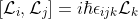(1)
Additionally, Schwabl , for example, defines the angular momentum operator, presents the commutation relations, and at least attempts (I think) to show (in a post-facto way) why they should have such relations. Likewise, in a related (as we'll see) problem, Goldstein, et. al.  discuss the commutation relations of generators of rotation without any physical argument.

However, both Sakurai  and Landau and Lifshitz , to some degree, present physical rationales for these relations. Landau and Lifshitz derive the notion of angular momentum in quantum theory quite nicely, and succinctly, but do not argue for why the commutation relations should hold. Sakurai develops a set of commutation relations independently of QM (as I will, shortly), but, I feel, bridges the gap to angular momentum rather poorly.

This post assumes familiarity with the ``generator of transformation'' ideas in .

The Generator of Rotation(a) In 3D.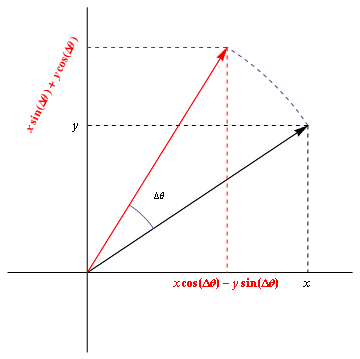(b) The projection of (a) onto the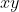-plane.
Figure 1: The rotation of a vector around the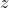-axis.

In a previous post I covered the notion of ``generators of transformations,'' and claimed, as an example, that the ``generator of rotation'' is the angular momentum. Actually, I was getting ahead of myself there, and the statement in that context was not entirely correct. As I did not derive this result in that post, I will now, and will hopefully clear things up.

Suppose we have a function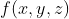and we want to rotate it in space around theaxis through some angle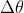to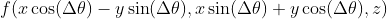. To do this, we'll find an ``angle rotation'' operator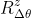, which, when applied to, gives. That is,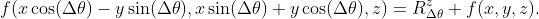(2)
The shift in coordinates can be derived from regular vector analysis, see Fig. 1 and Ref. , applied inside the arguments of the function.

Now the tricky part -- the Taylor expansion. Unlike the last time where the translated function had a simple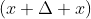argument, here we haveinside sines and cosines. Since I'm really too lazy to do this expansion by hand I had Mathematica do it for me (click to see full-size):
In Mathematica's notation,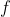raised to those parenthetical powers denotes partial derivatives. Say,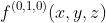means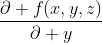, for example. This expression is a bit of a mess, but we are not completely lost. From our discussion in the beginning of , we know that at least one similar operator takes an exponential form. So, we'll guess that here, as well, our operator will take an exponential form. We just need to process the mess of (3) to find that hidden exponential.

The first two terms in the series give us hope. They can be written as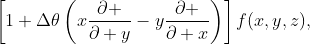(4)
which are, indeed, what we would expect to see at the beginning of an exponential expansion, where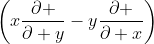is the generator. Now we check that this keeps up for higher powers.

Continuing with the quadratic term, let's see if we can write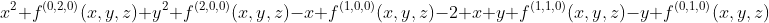as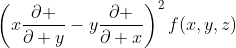which would be the next term in an exponential. We check: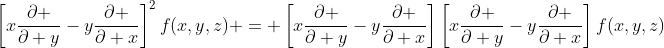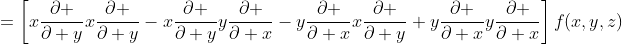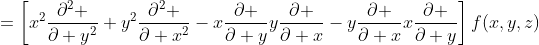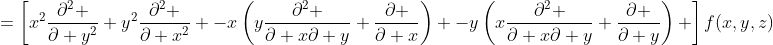(5)
which does match the mess for the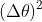term in the expansion (3). You can verify on your own that this pattern continues in the higher powers.

Thus we conclude that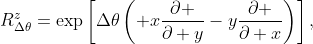(6)
where we now identify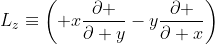(7)
as the generator of the rotation.

This generator is the-component of the cross product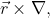where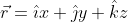and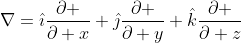. Thus, we can simplify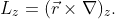(8)
If we carry through these same calculations for rotations around the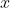or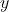axes (try it yourself!) we get similar generators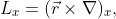(9)(10)
This allows us to write the rotation operator for a rotation around an arbitrary axis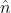, as(11)
where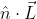for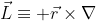(12)
is the generator of the transformation.

Commutators in general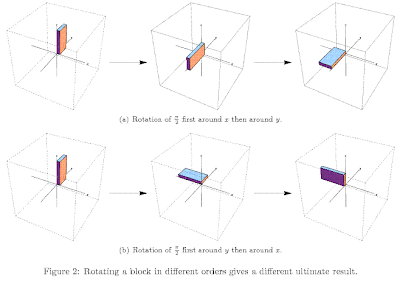In general, rotations do not commute. That is, rotating an object first around the-axis and then around the-axis will give a different result than rotating in the opposite order. You can convince yourself of this by the ultimate hand-waving argument2 -- twist your hand around different axes in different orders. Or see Fig. 2.

We'd like to find a way to quantify the difference between applying the rotations in different order, but, for the sake of generality, we'll discuss this for any two arbitrary operators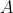and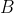. The most natural way to quantify a difference is to look at, well, the difference. That is, if these operators act on a vector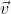, we'd like to know what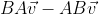(13)
is. This difference (for linear operators) does not depend on the particular vector, so we'll define the commutator of two operators as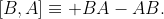(14)
Thus, a commutator of two operators is another operator which enacts this difference. If the order of operator application does not affect the end result the commutator is 0, and the operators are said to ``commute.''

In quantum mechanics, the issue of non-commuting operators is closely tied to the problem of measurement and the uncertainty principle. For example, if I have a state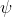and I want to measure the position I apply the position operator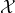. Likewise, if I want to measure the momentum I apply the momentum operator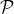. However, in quantum mechanics, the order of taking these measurements affects the results, such that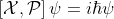, for example. However, the applicability of commutators is not relegated only to quantum mechanics.

Commutators for rotation
This brings us back to our original question of the commutator of rotations. Because any two rotations through arbitrary angles, done in opposite orders give drastically different results depending on the angles, we'll consider rotations through small angles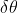, such that we can approximate (11) by the first two terms in the expansion: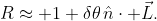(15)
This simpler expression makes calculating the commutator much simpler. For rotations around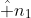and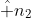, the commutator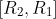depends only on the commutator of the generators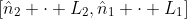.3 This commutator is the generator of the transformation for ``the difference between the order of the rotations.'' That is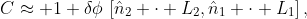(16)
where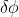is the parameter for this transformation. Then, just as any rotation can then be built up from repeated applications of the generator (as in that exponential), the commutators for larger angles can be built up from repeated applications of the commutators of the generators.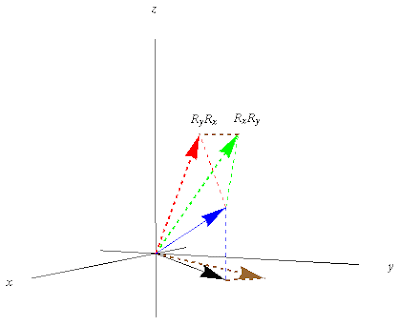Figure 3: Graphical commutator of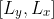. Blue vector is application of either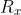or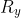. Red is further application oftoand green is further application ofto. Brown is difference between the two.

For ease of illustration, we'll consider small rotations around the- and-axes (i.e.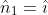and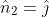). There are two ways to find the commutator. One way is by brute force calculation which I encourage you to try on your own (use the expressions for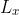(9) and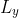(10)). However, I prefer showing it graphically, see Fig. 3. Starting with a vector in the-plane, we apply a small rotation around. This directs the vector upwards (blue in the picture). Then we apply another small rotation around, which directs the vector along the red line.

If we start with the same vector, and apply a small rotation around, the vector follows the blue line again. However, when we then rotate around, the vector veers off in the opposite direction at the same rate. The difference between the red and green vectors, as well as that difference added to the initial vector is shown in brown. The picture illustrates that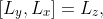(17)
i.e. the generator of rotation around the-axis. Similar relationships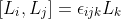(18)
hold for other permutations of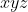.

Angular momentum
Looking back at the expression for the generator of rotations (12), we see that we can re-write this in terms of the momentum operator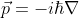(19)
in quantum mechanics: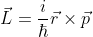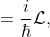(20)
where we call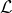the ``quantum mechanical angular momentum'' operator.4 Flipping this around to solve forin terms of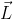: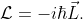(21)
In other words, the quantum mechanical angular momentum is the same (up to a constant) as the generator of rotations. Thus, the reason that quantum angular momentum has commutation relations (1) is due to the fact that it's simply a generator of rotation masquerading as a quantum mechanical operator.

References
 D.J. Griffths. Introduction to Electrodynamics. Pearson Prentice Hall, 3rd edition, 1999.
 F. Schwabl. Quantum Mechanics. Springer, 3rd edition, 2005.
 H. Goldstein, C. Poole, and J. Safko. Classical Mechanics. Cambridge University Press, San Francisco, CA, 3rd edition, 2002.
 J.J. Sakurai. Modern Quantum Mechanics. Addison-Wesley, San Francisco, CA, revised edition, 1993.
 L.D. Landau and E.M. Lifshitz. Quantum Mechanics. Butterworth-Heinemann, Oxford, UK, 3rd edition, 1977.
 E. Lansey. The Schrodinger Equation -- Corrections [online]. June 2009. Available from: http://behindtheguesses.blogspot.com/2009/06/schrodinger-equation-corrections.html.
 D.C. Lay. Linear Algebra and Its Applications. Addison-Wesley, Reading, MA, 3rd edition, 2003.
 C.T.J. Dodson and T. Poston. Tensor Geometry: The Geometric Viewpoint and its Uses. Springer, 2nd edition, 1997.

1 Everyone congratulate him on the birth of a son!
2 Borrowing a joke from Dodson and Poston, 
3 If this isn't obvious, work it out for yourself. Hint: The identity operator 1 commutes with everything.
4 There are better arguments (see ) using symmetry for whyshould actually be the angular momentum, not just called it, as I've argued, but they require much more talking. And this post is long enough already.